## Friday, March 30, 2018

### Excel and L1-norms

In an Excel spreadsheet I am studying (actually generating an optimization model from the formulas in the spreadsheet) I see the following construct:

=SUMIF(X,">0")-SUMIF(X,"<0")
where X is a range. This looks like a strange construct. It is meant to implement the sum of absolute values$||x||_1=\sum_i |x_i|$ also known as the L1-norm. The L2-norm $||x||_2 = \sqrt{\sum_i x_i^2}$ is easy to do in Excel:

=SQRT(SUMSQ(X))

Strangely, there is no obvious (that is, obvious to me) formula to do the L1-norm. Excel is a large program, with tons of options: there is almost always some workaround available. In  a list is provided.

The  L$$\infty$$-norm defined by$||x||_{\infty}=\max_i |x_i|$has similar issues: there is no built-in formula for this.

Here is summary:

The =SUM(ABS(X)) and =MAX(ABS(X)) formulas do not give what we want. It is somewhat surprising that =SUMPRODUCT(ABS(X)) is doing the right thing (although the name of the function is now misleading: we dropped the PRODUCT part of the functionality). The array formulas  seem to be most intuitive.

## Monday, March 26, 2018

### MIP Gap Artifacts

For large integer programming models we may not be always willing to wait for a global, proven optimal solution. A straightforward way to deal with this is to set a time limit. A different way is to set a gap tolerance on the gap between the best possible and the best found integer solution. A typical gap tolerance is a few percent. Integer solvers display the gap prominently in the log. In most cases we talk about a relative gap. There is also an absolute gap with a corresponding absolute gap tolerance. In the following I talk about the relative gap.

It may come as a surprise, but the MIP gap can increase. From a Cplex log:

        Nodes                                         Cuts/
Node  Left     Objective  IInf  Best Integer    Best Bound    ItCnt     Gap

*     0+    0                          300.0000     -120.0000           140.00%
Found incumbent of value 300.000000 after 0.09 sec. (5.80 ticks)
0     0     -120.0000   153      300.0000     -120.0000      229  140.00%
0     0     -120.0000   163      300.0000     Cuts: 118      356  140.00%
0     0     -120.0000   129      300.0000      Cuts: 64      427  140.00%
0     0     -120.0000   142      300.0000     Cuts: 180      503  140.00%
*     0+    0                          -10.0000     -120.0000              ---
Found incumbent of value -10.000000 after 0.31 sec. (67.99 ticks)
0     2     -120.0000   128      -10.0000     -120.0000      503     ---
Elapsed time = 0.33 sec. (71.72 ticks, tree = 0.01 MB, solutions = 2)
414   350     -120.0000   155      -10.0000     -120.0000     8074     ---
Cuts: 60
*   500+  375                          -30.0000     -120.0000           300.00%
Cuts: 4
Found incumbent of value -30.000000 after 1.44 sec. (418.43 ticks)
500   377     -110.0000   148      -30.0000     -120.0000     9855  300.00%
610   483     -120.0000   157      -30.0000     -120.0000    12092  300.00%
632   376     -120.0000   176      -30.0000     -120.0000    12662  300.00%
710   268     -110.0000   166      -30.0000     -120.0000    13622  300.00%
950   360      -73.0094   112      -30.0000     -120.0000    16832  300.00%
Cuts: 216
1110   430      -89.6733   148      -30.0000     -120.0000    19495  300.00%
1398   650      -91.0030   118      -30.0000     -120.0000    25098  300.00%
Cuts: 72
1759   958      -43.2768    95      -30.0000     -120.0000    31198  300.00%
3031  2036      -60.1740    97      -30.0000     -119.2262    49706  297.42%
Elapsed time = 13.67 sec. (4413.94 ticks, tree = 0.73 MB, solutions = 3)
4721  3471      -77.9342   132      -30.0000     -116.6542    71843  288.85%
Cuts: 42
5867  4399      -82.3794   152      -30.0000     -115.2913    89434  284.30%
Cuts: 8
7276  5520      -95.5742   137      -30.0000     -113.0523   107572  276.84%
Cuts: 20
8729  6670      -68.9411   119      -30.0000     -110.8877   127085  269.63%
Cuts: 28
9919  7614      -40.0000    86      -30.0000     -110.0000   145286  266.67%


The reason is the Best Integer bound assumes vary small values when passing the x-axis.

#### GAP definitions

The Cplex definition of the relative gap is :
$\mathit{RelGap} = \frac{|\mathit{BestBound}-\mathit{BestInteger}|}{10^{-10}+ |\mathit{BestInteger}|}$

This means the following scenario is possible:

Although he absolute gap (the difference between the grey and the orange line) is decreasing monotonically, the relative gap (the yellow line) is not. Note that the gap relates to the scale on the right (with percentages). Just to be sure: the horizontal axis is time.

Often the BestBound and the BestInteger quantities are called the Lower and Upper Bound. (Note: this makes sense when minimizing. If maximizing we need to flip these.) So we can write the Cplex relative gap as (approximately):
$\mathit{RelGap} = \frac{|U-L|}{ |U|}$

Most solvers use a similar relative gap. Some solvers such as Baron  use a slightly different definition:
$\mathit{RelGap} = \frac{|U-L|}{ |L|}$

This can make a difference. When using bounds in the picture, the Baron relative gap would look like:

In this case the Baron relative gaps are better than the ones for Cplex. This is not always the case. Below is a Baron log:

  Iteration    Open nodes         Time (s)    Lower bound      Upper bound
*         1             1             4.00      0.00000         10.0000
1             1             4.00     0.376100E-01     10.0000
12+            6            34.00     2.50000          10.0000
19+           11            65.00     2.55875          10.0000
29+           14            95.00     2.55875          10.0000
40+           18           125.00     2.55875          10.0000
51+           19           156.00     2.56067          10.0000
59+           17           186.00     2.73249          10.0000
70+           17           217.00     3.32720          10.0000
81+           14           247.00     4.10826          10.0000
88+           14           277.00     5.00000          10.0000
100+           10           308.00     5.15290          10.0000
111+            6           338.00     5.51454          10.0000
123+            4           368.00     5.98316          10.0000
129             0           382.00     9.09091          10.0000


If we calculate both relative gaps for these bounds we see:

Here the Baron gap is more difficult.

GAMS suggests an even more crazy formula :
$\mathit{RelGap} = \frac{|U-L|}{\max( |U|,|L|)}$

Finally returning to our first Cplex log. We see very large gaps. If you are embarrassed about these large gaps, you can easily reformulate the objective. After using $\min\> z = 1000 + z_{\text{orig}}$ we see:

        Nodes                                         Cuts/
Node  Left     Objective  IInf  Best Integer    Best Bound    ItCnt     Gap

*     0+    0                         1300.0000      880.0000            32.31%
Found incumbent of value 1300.000000 after 0.08 sec. (5.85 ticks)
0     0      880.0000   153     1300.0000      880.0000      229   32.31%
0     0      880.0000   163     1300.0000     Cuts: 118      356   32.31%
0     0      880.0000   129     1300.0000      Cuts: 64      427   32.31%
0     0      880.0000   142     1300.0000     Cuts: 180      503   32.31%
*     0+    0                          990.0000      880.0000            11.11%
Found incumbent of value 990.000000 after 0.41 sec. (68.34 ticks)
0     2      880.0000   128      990.0000      880.0000      503   11.11%
Elapsed time = 0.80 sec. (72.31 ticks, tree = 0.01 MB, solutions = 2)
2579  2059      923.8533    99      990.0000      880.0000    31891   11.11%
Cuts: 78
*  2581+ 1437                          980.0000      880.0000            10.20%
Found incumbent of value 980.000000 after 2.05 sec. (311.11 ticks)


So just looking at the relative gap in isolation is not that useful: we can always fudge it.

#### References

1. Cplex Relative MIP gap tolerance, https://www.ibm.com/support/knowledgecenter/SSSA5P_12.8.0/ilog.odms.cplex.help/CPLEX/Parameters/topics/EpGap.html
3. What is optca/optcr? https://support.gams.com/solver:what_is_optca_optcr

## Sunday, March 18, 2018

### Piecewise linear regression

In  a data set is presented, where a piecewise or segmented linear regression approach can help. A linear fit looks like:

There are two issues we can see from the plot: we can expect a better fit if we can use a piecewise linear function with 2 segments. Secondly the standard regression will allow negative predictions for $$\hat{Y}$$ when we have small $$X$$ values.

The breakpoint is estimated to be $$\bar(x)=1.5$$. The plots seem convincing.

These results were produced using SAS.

Let's see what happens if we use some more advanced mathematical optimization models. The linear model is easily coded as QP problem:

\begin{align} \min \>& \text{SSQ} = \sum_i e_i^2 \\ & y_i = a + b x_i + e_i \end{align} where $$x,y$$ are data.

This purely linear fit is of course identical:

Now let's see if we can tackle a piecewise linear function with two segments. For this we introduce a binary variable $$\delta_i \in \{0,1\}$$ to indicate if an observation belongs to the first or the second segment. A high-level model can look like:

\begin{align} \min & \>\text{SSQ} = \sum_i e_i^2 && (1)\\ & e_i = (1-\delta_i) r_{1,i} + \delta_i r_{2,i} &&(2)\\ &r_{k,i} = y_i - a_k - b_k x_i && (3)\\ & x_i \le \bar{x} \Rightarrow \delta_i = 0 &&(4)\\& x_i \gt \bar{x} \Rightarrow \delta_i = 1 && (5)\\ & \bar{y} = a_k + b_k \bar{x}&& (6)\end{align}

Here $$(\bar{x}, \bar{y})$$ is the break point (to be determined by the model).

If we delete the last constraint, we can formulate the problem as a convex MIQP. This requires linearization of the constraints (2), (4) and (5).

Adding the last constraint $$\bar{y} = a_k + b_k \bar{x}$$ gives us a non-convex MINLP. After solving it we see:

Interestingly these results are very different from . A more formal optimization model can make a lot of difference compared to a more ad-hoc method. The pictures from  indicate we are not optimal with respect to the total Sum of Squares. The continuous version of the segmented model with a breakpoint at 1.68 as suggested in  has: SSQ=0.00235. This is larger than our SSQ=0.00206459 with a breakpoint at 1.8135.

The two rightmost observations are probably outliers that should be removed from the model.

#### Update: Alternative formulations

If the $$x_i$$ are sorted: $$x_{i+1} \ge x_i$$, we can replace the implications
\begin{align} & x_i \le \bar{x} \Rightarrow \delta_i = 0 &&(4)\\& x_i \gt \bar{x} \Rightarrow \delta_i = 1 && (5) \end{align}  by $\delta_{i+1} \ge \delta_{i}$ I.e. we switch once from 0 to 1, Of course the sorting is no problem: we can sort the data before doing the optimization.

If furthermore we restrict the breakpoint $$\bar{x}$$ to one of the data points, additional reformulations are possible. See the comments below. This can make the model convex. I think for the problem at hand we can add this restriction without much impact on the sum of squares. Also this may be a very good model to solve first: it will be an excellent starting point for the non-convex model. (I used the discontinuous version to generate a good starting point for the non-convex MINLP, but surely this version must be better).

In  the problem is stated as the regression: $y = a + b x + c (x-\bar{x}) _{\mathbf{+}}$ where $$x_{\mathbf{+}} = \max(x,0)$$. $$c$$ is now the difference in slope for the second segment. This leads to a simpler optimization problem. The term $$x-\bar{x}$$ can be linearized using a big-M formulation. The model can look like: \begin{align} \min \>& \sum_i e_i^2 \\ & y_i = a + b x_i + c z_i + e_i\\ & z_i = \max(x_i-\bar{x},0) \end{align} As we have a multiplication of two variables $$c z_i$$, we have not made much progress,

#### R package segmented

The R package segmented is actually doing slightly better. Like the previous Baron solution it puts the last two observations in to the second segment, but then moves $$\bar{x}$$ somewhat:

> lm.out <- lm(y~x)
> summary(lm.out)

Call:
lm(formula = y ~ x)

Residuals:
Min        1Q    Median        3Q       Max
-0.018152 -0.002764  0.000568  0.002367  0.035213

Coefficients:
Estimate Std. Error t value Pr(>|t|)
(Intercept) -0.013224   0.002454   -5.39 8.06e-07 ***
x            0.021558   0.002047   10.53 2.26e-16 ***
---
Signif. codes:  0 ‘***’ 0.001 ‘**’ 0.01 ‘*’ 0.05 ‘.’ 0.1 ‘ ’ 1

Residual standard error: 0.007519 on 74 degrees of freedom
Multiple R-squared:  0.5999, Adjusted R-squared:  0.5945
F-statistic:   111 on 1 and 74 DF,  p-value: 2.257e-16

> SSQ <- sum(lm.out$residuals^2) > SSQ  0.004183108 > seg.out<-segmented(lm.out,seg.Z = ~x) > summary(seg.out) ***Regression Model with Segmented Relationship(s)*** Call: segmented.lm(obj = lm.out, seg.Z = ~x) Estimated Break-Point(s): Est. St.Err 1.821 0.097 Meaningful coefficients of the linear terms: Estimate Std. Error t value Pr(>|t|) (Intercept) -0.008816 0.001826 -4.828 7.52e-06 *** x 0.016833 0.001564 10.763 < 2e-16 *** U1.x 0.152178 0.063636 2.391 NA --- Signif. codes: 0 ‘***’ 0.001 ‘**’ 0.01 ‘*’ 0.05 ‘.’ 0.1 ‘ ’ 1 Residual standard error: 0.005359 on 72 degrees of freedom Multiple R-Squared: 0.8022, Adjusted R-squared: 0.794 Convergence attained in 4 iterations with relative change 2.835799e-16 > SSQ2 <- sum(seg.out$residuals^2)
> SSQ2
 0.00206802


This is very close to what Baron produced (Baron is just a slightly bit better).

#### References

1. Sandra E. Ryan; Laurie S. Porth, A tutorial on the piecewise regression approach applied to bedload transport data, Gen. Tech. Rep. RMRS-GTR-189. Fort Collins, CO: U.S. Department of Agriculture, Forest Service, https://www.fs.fed.us/rm/pubs/rmrs_gtr189.pdf
2. Muggeo, V.M.R. (2008) Segmented: an R package to fit regression models with broken-line relationships. R News 8/1, 20–25.

## Wednesday, March 14, 2018

### production scheduling: Minimum up-time

In  a minimum up-time question is posted:

• Assume we have $$T=12$$ periods. If we produce in period $$t$$ we have a profit of $$c_t$$. Some $$c_t$$'s are negative.
• If we turn production on, it should remain on for at least $$n=5$$ periods.
• We want to maximize total profit.
• How can we write a constraint that captures this minimum up-time condition?

We can recognize production is turned on in period $$t$$ by observing $$x_{t-1}=0$$ and $$x_t=1$$ where $$x_t$$ is a binary variable indicating whether our production process is turned on or off. So our constraint can look like:
$x_{t-1}=0,x_{t}=1 \Longrightarrow \sum_{k=t}^{t+n-1} x_k = n\>\>\>\forall t$
In a MIP model we can translate this into:
$\sum_{k=t}^{t+n-1} x_k \ge n(x_t-x_{t-1}) \>\>\>\forall t$
This formulation is not completely obvious. We note that $$x_t-x_{t-1}$$ is zero or negative for all cases except $$x_{t-1}=0,x_{t}=1$$.

Some notes:
• Behavior near the boundaries is always important. E.g. we could assume $$x_0=0$$.
• Let's also assume $$x_{T+1}=0$$ so we need to finish our production run in the time horizon.
• The MIP constraint can also be written as: $\sum_{k=t+1}^{t+n-1} x_k \ge (n-1)(x_t-x_{t-1}) \>\>\>\forall t$ as we know $$x_t=1$$ when this thing kicks in.
• So this gives us 12 constraints for our $$T=12, n=5$$ problem.
• We can disaggregate the summation into individual constraints $x_k \ge x_t-x_{t-1}\text{ for } k=t+1,\dots,t+n-1$ This is tighter and may solve faster. Advanced solvers may use an "implied bound" cut to do this automatically (and smarter) .
A complete model can look like:
\begin{align} \max \>&\sum_t c_t x_t\\ &\sum_{k=t}^{t+n-1} x_k \ge n(x_t-x_{t-1}) \\ & x_t \in \{0,1\}\end{align}
I implemented this in GAMS. GAMS assumes that variables indexed outside their domain are zero. This means I don't have to do anything special for $$t=1$$ and for $$t\gt T-n$$.

A solution with random $$c_t$$ is: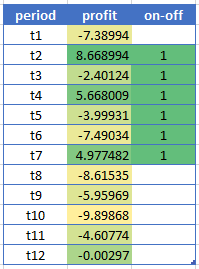Optimal Solution
For this small case we could have enumerated all possible feasible production schedules and pick the best one. We have 42 possible solutions: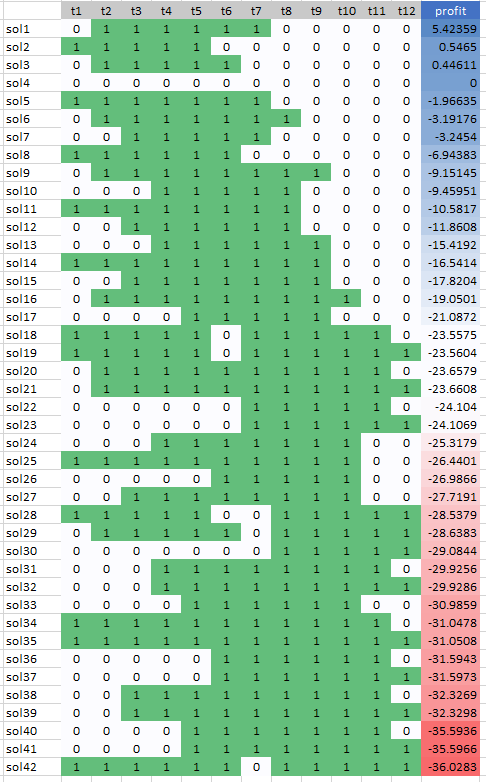All Feasible Solutions ordered by Total Profit

For $$T=24,n=5$$ we have 24 constraints, while enumeration of all feasible solutions would lead to 4316 solutions. NB: I used the Cplex solution pool to enumerate the feasible solutions.Optimal solution for T=24

There are a number of extensions one can encounter:

• There may be some initial state. We need more than just the state at $$t=0$$. Basically we need to know for how many periods we are in the 'on'-state.
• We may relax the assumption that $$x_{T+1}=0$$.
• A maximum up-time is also not too difficult using a similar construct. Say we limit up-time to $$m$$ periods. Then we can require:$\sum_{k=t}^{t+m} x_k \le m$ I.e. we don't want to see a series of $$m+1$$ ones. See the picture below for an example with $$T=24, n=3, m=4$$.
• A minimum down-time is sometimes encountered in power generation models. This is close to minimum up-time: make $$y_t=1-x_t$$ and apply our tools we described above on $$y_t$$.
• Limiting the number of start-ups or shut-downs is quite a standard requirement. E.g. to limit the number of times we start a production run: \begin{align} &s_t \ge x_t-x_{t-1}\\& \sum_t s_t \le K \\ & s_t \in \{0,1\} \end{align}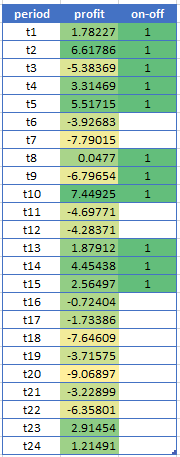Run-lengths between 3 and 5.

#### Nitty-Gritty Implementation Details

The basic constraints discussed above are: \begin{align}&\sum_{k=t}^{t+n-1} x_k \ge n(x_t-x_{t-1}) &\forall t &\text{ (minimum length)}\\ & \sum_{k=t}^{t+m} x_k \le m & \forall t & \text{ (maximum length)} \end{align}  In GAMS, the minimum and maximum run-length constraints can be stated straightforwardly as:

alias(t,k);

minlength(t)..

sum(k$(ord(k)>=ord(t) and ord(k)<=ord(t)+n-1), x(k)) =g= n*(x(t)-x(t-1)); maxlength(t).. sum(k$(ord(k)>=ord(t) and ord(k)<=ord(t)+m), x(k)) =l= m;

I often prefer to keep model equations as simple as possible. We can do this by precalculating a set tk(t,k):

set tk(t,k) 'for use in minlength';
tk(t,k) =
ord(k)>=ord(t) and ord(k)<=ord(t)+n-1;
display tk;

minlength(t)..

sum(tk(t,k), x(k)) =g= n*(x(t)-x(t-1));Structure of the set tk

This also allows us to debug things before the rest of the model is complete. We can simply use display statements or use the gdx viewer. Equations can only be meaningfully debugged by trying to solve the model they are part of.

A slightly different approach is to rely on leads:\begin{align}&\sum_{k=1}^{n} x_{t+k-1} \ge n(x_t-x_{t-1}) &\forall t &\text{ (minimum length)}\\ & \sum_{k=1}^{m+1} x_{t+k-1} \le m & \forall t & \text{ (maximum length)} \end{align}This is also easily transscribed into GAMS syntax:

minlength(t)..

sum(k$(ord(k)<=n), x[t+(ord(k)-1)]) =g= n*(x(t)-x(t-1)); maxlength(t).. sum(k$(ord(k)<=m+1), x[t+(ord(k)-1)] ) =l= m;

#### More details: disaggregated version

The disaggregated version$x_k \ge x_t-x_{t-1}\text{ for } k=t+1,\dots,t+n-1$ needs a little bit more attention.  If we just translate this to:

minlength2(tk(t,k))..
x(k) =g= x(t)-x(t-1);

then we allow partial runs near the end of the planning horizon.Partial runs are not allowed

To prevent this we need to forbid $$x_t \gt x_{t-1}$$ near the end.

minlength2a(tk(t,k))..
x(k) =g= x(t)-x(t-1);

minlength2b(t)$( ord(t)+n-1 > card(t)).. x(t) =l= x(t-1); Interestingly (at least to me), the "leads" formulation is easier. We just can use: minlength3(t,k)$(ord(k)<=n-1)..
x(t+
ord(k)) =g= x(t)-x(t-1);

This will automatically generate $$0 \le x_t-x_{t-1}$$ where needed.

To get a feeling about possible performance improvement when disaggregating the min-length constraint, we need a slightly larger problem. So I used $$T=1024$$ and used $$N=10$$ machines. So our decision variable is now $$x_{i,t}$$.

AggregatedDisaggregated
Size
SINGLE EQUATIONS       10,241
SINGLE VARIABLES       10,241
DISCRETE VARIABLES     10,240

SINGLE EQUATIONS       40,961
SINGLE VARIABLES       10,241
DISCRETE VARIABLES     10,240

Objective 17304.883540 17304.883540
Iterations, Nodes 10547 iterations, 13 nodes 6234 iterations, 0 nodes
Time Cplex Time: 7.39sec (det. 1900.93 ticks) Cplex Time: 5.13sec (det. 1728.88 ticks)

The disaggregated version does better: it can solve the problem during preprocessing.

#### The quest for zero nodes

After doing these experiments with disaggregation I was asking myself:

Is there an aggregated formulation that solves this problem in zero nodes?

It is standard wisdom in integer programming that one should disaggregate variable upper bound constraints on sums of variables. These are constraints of the form:$x_1+\dots+x_n \le (u_1+\dots+u_n) y, \> y \in \{0,1\}$where $$u_j$$ is a valid upper bound on $$x_j \ge 0, (j = 1,\dots,n)$$. This single constraint is equivalent, given the integrality of $$y$$, to the following collection of "disaggregated" constraints: $x_j \le u_j y_j \>(j = 1,\dots,n)$The reason the second, disaggregated formulation is preferred is that, while equivalent given integrality, its linear-programming relaxation is stronger. However, given the ability to automatically disaggregate the first constraint, these "implied bound" constraints can be stored in a pool and added to the LP only as needed. Where $$n$$ is large this latter approach will typically produce a much smaller, but equally effective LP.
Our constraint $\sum_{k=t}^{t+n-1} x_k \ge n(x_t-x_{t-1}) \> \forall t$ is not exactly this form. We can coerce this by:\begin{align} & \sum_{k=t}^{t+n-1} x_k \ge n y_t\\ & y_t \ge x_t-x_{t-1}\\ &y_t \in \{0,1\}\end{align} Here are the results:

AggregatedDisaggregatedAggregated v2
SINGLE EQUATIONS       10,241
SINGLE VARIABLES       10,241
DISCRETE VARIABLES     10,240

SINGLE EQUATIONS       40,961
SINGLE VARIABLES       10,241
DISCRETE VARIABLES     10,240

SINGLE EQUATIONS       20,481
SINGLE VARIABLES       20,481
DISCRETE VARIABLES     20,480

obj=17304.883540 obj=17304.883540 obj=17304.883540
10547 iterations, 13 nodes 6234 iterations, 0 nodes 12477 iterations, 0 nodes
Cplex Time: 7.39sec (det. 1900.93 ticks) Cplex Time: 5.13sec (det. 1728.88 ticks) Cplex Time: 20.61sec (det. 6098.72 ticks)

We achieved our zero nodes, but at a price that is too high. In any case the Cplex performance on this model (with any of these formulations) is fantastic.

#### References

1. Linear programming - optimizing profit with tricky constraints, https://stackoverflow.com/questions/49261669/linear-programming-optimizing-profit-with-tricky-constraints
2. Bixby E.R., Fenelon M., Gu Z., Rothberg E., Wunderling R. (2000) MIP: Theory and Practice — Closing the Gap. In: Powell M.J.D., Scholtes S. (eds) System Modelling and Optimization. CSMO 1999. IFIP — The International Federation for Information Processing, vol 46. Springer, Boston, MA

## Sunday, March 11, 2018

### Regular Constraints

Here is an interesting problem (1) that can be stated in terms of regular expressions.

#### Problem

Puzzle description: The player is given an empty game board (varying size) that they must fill with n-colors, using clue patterns for the rows and columns. Each clue pattern is the sequence of colors in that row/col but with consecutive duplicates removed.

We can also write the hints using regular expressions. E.g. the first row would have r+g+b+.

#### Constraint programming

Constraint solvers like Minizinc/Gecode has a regular constraint . Not for the fainthearted: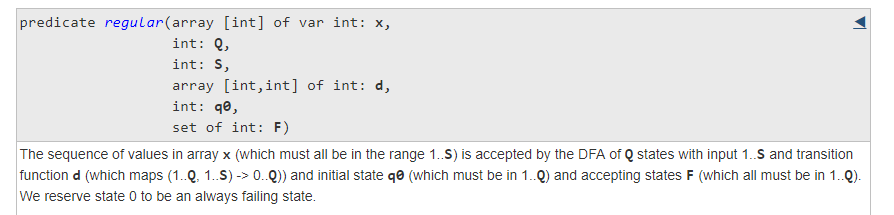Regular Constraint in Minizinc
This is what a regular expression algorithm will do in the background: execute a state machine.

Let's see how we can model the first row with this. Obviously the pattern rgb allows for the following solutions: "rrgb", "rggb", "rgbb". We encode the colors with integers. The decisions variables $$x$$ are now just an array of integers between $$1 \le x_i \le nc$$ where $$nc$$ is the number of colors. This small problem can be encoded in Minizinc as:

include "globals.mzn";

int: n = 4;    % length of row
int: nc = 3;   % number of colors

array[1..n] of var 1..nc: x;

int: q = 4; % number of states
int: q0 = 1;  % initial state
set of int: q1 = {4}; % final state
array[1..q,1..nc] of int: p =
[|
%  r  g  b
2, 0, 0 |   % 1: accept r
2, 3, 0 |   % 2: accept r or g
0, 3, 4 |   % 3: accept g or b
0, 0, 4 |   % 4: accept b
|];

constraint regular(x, q, nc, p, q0,  q1);

solve satisfy;

output [show(x)];


The state transition table p is often depicted as a graph. In this case it is very simple:

The solution with Minizinc/Gecode looks like: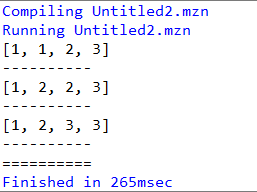Possible solutions for first row

where $$1=r, 2=g, 3=b$$.

This can be repeated for each row and column with non-trivial patterns. There must be a better way to do this, but here is my attempt:

include "globals.mzn";

int: m = 4;    % number of rows
int: n = 4;    % length of columns
int: nc = 3;   % number of colors

% r = 1
% g = 2
% b = 3

array[1..m,1..n] of var 1..nc: x;

int: q = 4; % number of states
int: q0 = 1;  % initial state
set of int: q1 = {4}; % final state
array[1..q,1..nc] of int: p_rgb =
[|
%  r  g  b
2, 0, 0 |   % 1: accept r
2, 3, 0 |   % 2: accept r or g
0, 3, 4 |   % 3: accept g or b
0, 0, 4 |   % 4: accept b
|];

array[1..q,1..nc] of int: p_brg =
[|
%  r  g  b
0, 0, 2 |   % 1: accept b
3, 0, 2 |   % 2: accept b or r
3, 4, 0 |   % 3: accept r or g
0, 4, 0 |   % 4: accept g
|];

array[1..q,1..nc] of int: p_rbg =
[|
%  r  g  b
2, 0, 0 |   % 1: accept r
2, 0, 3 |   % 2: accept r or b
0, 4, 3 |   % 3: accept b or g
0, 4, 0 |   % 4: accept g
|];

array[1..q,1..nc] of int: p_rbr =
[|
%  r  g  b
2, 0, 0 |   % 1: accept r
2, 0, 3 |   % 2: accept r or b
4, 0, 3 |   % 3: accept b or r
4, 0, 0 |   % 4: accept r
|];

array[1..q,1..nc] of int: p_grb =
[|
%  r  g  b
0, 2, 0 |   % 1: accept g
3, 2, 0 |   % 2: accept g or r
3, 0, 4 |   % 3: accept r or b
0, 0, 4 |   % 4: accept b
|];

%---- row 1
constraint regular(row(x, 1), q, nc, p_rgb, q0, q1);

%---- row 2
constraint regular(row(x, 2), q, nc, p_brg, q0, q1);

%---- row 3
constraint row(x, 3) = [3,3,3,3];

%---- row 4
constraint row(x, 4) = [2,1,3,2];

%---- col 1
constraint regular(col(x, 1), q, nc, p_rbg, q0, q1);

%---- col 2
constraint regular(col(x, 2), q, nc, p_rbr, q0, q1);

%---- col 3
constraint regular(col(x, 3), q, nc, p_grb, q0, q1);

%---- col 4
constraint col(x, 4) = [3,2,3,2];

solve satisfy;

output [show(x)];


Notice that all the p matrices are essentially column permuted versions of each other. The results are: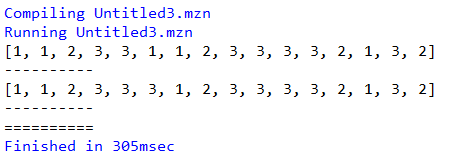Solution with Minizinc/Gecode
Too bad Minizinc is not printing the matrix in a nicer way, but with some effort we can see this is correct.

#### MIP Formulation

Inside a MIP we only have linear equations to our disposal. One way to attack the above problem is to generate all possible "strings". I.e. we would have:

• Row 1 is "rrgb", "rggb" or "rgbb"
• Row 2 is "bbrg", "brrg" or "brgg"
• Row 3 is 'bbbb'
• Row 4 is 'grbg'
• Etc.
The model equations can look like:

Here rows(i,k,j,c)=true if the k-th version of row i has color c in column position j. The sets ik(i,k) and jk(j,k) contain the valid elements k for each row or column (rows and columns have different numbers of allowed patterns, e.g. row 3 has only one allowed pattern: 'bbbb').

After adding a cut to forbid previously found solutions and looping until the model becomes infeasible we get the two solutions:

----    104 PARAMETER sols  solutions

INDEX 1 = iter1

R           G           B

i1.j1       1.000
i1.j2       1.000
i1.j3                   1.000
i1.j4                               1.000
i2.j1                               1.000
i2.j2       1.000
i2.j3       1.000
i2.j4                   1.000
i3.j1                               1.000
i3.j2                               1.000
i3.j3                               1.000
i3.j4                               1.000
i4.j1                   1.000
i4.j2       1.000
i4.j3                               1.000
i4.j4                   1.000

INDEX 1 = iter2

R           G           B

i1.j1       1.000
i1.j2       1.000
i1.j3                   1.000
i1.j4                               1.000
i2.j1                               1.000
i2.j2                               1.000
i2.j3       1.000
i2.j4                   1.000
i3.j1                               1.000
i3.j2                               1.000
i3.j3                               1.000
i3.j4                               1.000
i4.j1                   1.000
i4.j2       1.000
i4.j3                               1.000
i4.j4                   1.000


Reference  gives a direct approach to represent the regular constraint in a MIP. I think this will be somewhat messy because we have multiple regular expressions in our model.

#### References

1. Constraint programming; Fill grid with colors following pattern rules, https://stackoverflow.com/questions/49101990/constraint-programming-fill-grid-with-colors-following-pattern-rules
2. Minizinc Documentation - Standard Library. Extensional constraints (table, regular etc.), http://www.minizinc.org/doc-lib/doc-globals-extensional.html
3. Côté MC., Gendron B., Rousseau LM. (2007) Modeling the Regular Constraint with Integer Programming. In: Van Hentenryck P., Wolsey L. (eds) Integration of AI and OR Techniques in Constraint Programming for Combinatorial Optimization Problems. CPAIOR 2007. Lecture Notes in Computer Science, vol 4510. Springer, Berlin, Heidelberg